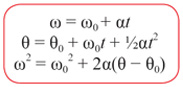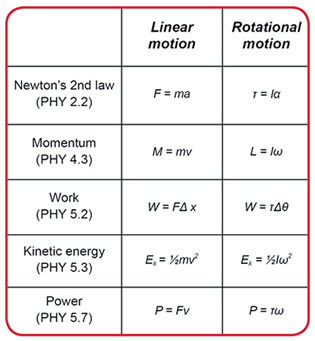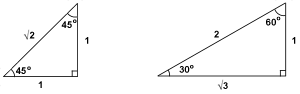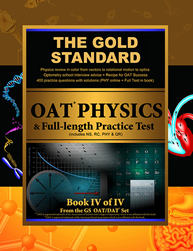[ Log In | Register | FAQ ]

# OAT Physics Equation Lists (Formulas)## OAT Physics Topics

Official (ASCO/ADA) OAT physics topics include the following: Units and vectors, linear kinematics, statics, dynamics, rotational motion, energy and momentum, simple harmonic motion: springs, period, angular frequency, waves and interference, fluid statics, thermal energy and thermodynamics, electrostatics, DC circuits: voltage, current, resistance, capacitors, transformers, magnetism, optics (mirrors and lenses), and modern physics (i.e. nuclear/atomic physics).

Be sure that you are familiar with the SI units for all topics that you review and, of course, how to interconvert SI units (i.e. joules to picojoules or kilojoules, etc.; force is in newtons and one newton is equal to one kilogram-meter per second squared, etc.). To make it easier for you to memorize OAT Physics equations, you should consider printing our suggested equation list below or making your own OAT equation formula sheet. Review your equations frequently leading up to the real exam. Of course, understanding is always better than just memorization.

OAT physics topics are not identical to MCAT topics. It is important to know the difference because some students prepare for the OAT using MCAT books and then are surprised when they see the real OAT exam. Note that the MCAT covers AC circuits and fluid dynamics (i.e. Bernouilli's equation, continuity) which are NOT OAT Physics topics. On the other hand, the OAT includes angular rotation equations which are not MCAT topics.

## OAT Physics Equations: Memorize These!

 alpha: α mu: μ delta: Δ

If you don't see the Greek symbols above then the equations below will not make sense; thus adjust fonts on your browser to Unicode.

 Translational motion x = x 0 + v 0 t + 1/2at2 Vƒ = Vo + at Frictional force f max = μ Ν μk < μs always Uniform circular motion Fc = mac = mv2 /r ac= v2 /r Momentum, Impulse I = F Δt = ΔM M = mv Work, Power W = F d cosθ P = ΔW/Δt Energy (conservation) ET = Ek + Ep E = mc2 Spring Force, Work F = -kx W = kx2 /2 Current and Resistance I = Q/t R = ρl/A Resistors (series, par.) Req = R1 + R2 . . . 1/ Req = 1/ R1 +1/ R2 Sound dB = 10 log 10 (I/I0 ) beats = Δ f Kirchoff's Laws Σi = 0 at a junction ΣΔV = 0 in a loop Thermodynamics Q = mc Δ T Q = mL Torque forces L1 = F1× r1 (CCW + ve) L2 = F2 × r2 (CW -ve) Torque force at EQ ΣFx = 0 and ΣFy = 0 ΣL = 0 Refraction ( sin θ1 )/(sin θ2 ) = v1 /v2 = n2 /n1 = λ1 /λ2 n = c/v 1/ Ceq = 1/ C1 + 1/ C2 + 1/ C3 . . . Capacitors in Ser. and Par. Ceq = C1 + C2 . . . Potential Energy ( PE ) = W = 1/2 QV Work in Electricity W = 1/2 CV2 V = Ed for a parallel plate capacitor d = the distance between the plates

## OAT Physics Equations: Memorize as Pairs

 F = ma F = qE Similar Form F = KG ( m1 m2 / r2 ) F = k ( q1 q2 / r2 ) V = IR P = IV Paired Use vav = Δ d / Δ t aav = Δ v / Δ t (avg vel, acc) v = λ f E = hf (f = 1/T) Ek = 1/2 mv2 Ep = mgh (kin, pot E) Ρ = F/A Δ Ρ = ρgΔh (pressure Ρ) SG = ρ substance / ρ water ρ = 1 g/cm3 = 103 kg/m3 (Spec Grav) ρ = mass / volume Fb = Vρg = mg (buoyant F) 1/ i + 1/ o = 1/ f = 2/r = Power M = magnification = - i/o Optics Note: Specific gravity (SG) is equivalent to the fraction of the height of a buoyant object below the surface of the fluid.

Linear motion Rotational motion
Force Torque
Mass Moment of inertia
Distance Traveled
(displacement)
Angle rotated
(angular displacement)
Velocity Angular velocity
Acceleration Angular acceleration
Momentum Angular momentum## Don't Memorize, Know How to Use...

 L = L0 (1 + αΔ T ) Linear Expansion Solids, Temp Δ A = A0(1 + γΔ T ) Area Expansion V = V0(1 + βΔ T ) Volume Expansion β = 3 α fo = fs (V ± vo )/( V ± vs ) Doppler Effect: when d is decreasing use + vo and - vs dF = dq v(B sin α) = I dl(B sin α) Laplace's Law RH rule

## Atomic Physics

• alpha (α) particle = 2He4 (helium nucleus);
• beta (β) particle = -1e0 (an electron);
• a positron +1e0 (same mass as an electron but opposite charge);
• gamma (γ) ray = no mass, no charge, just electromagnetic energy;
• Δ m /Δ t = rate of decay where Δ m = change in mass, Δ t = change in time.
• If the number of half-lifes n are known we can calculate the percentage of a pure radioactive sample left after undergoing decay since the fraction remaining = (1/2) n.

Nelectrons = 2 n2 , where Nelectrons designates the number of electrons in shell n .

The state of each electron is determined by the four quantum numbers:

• principal quantum number n determines the number of shells, possible values are: 1 (K), 2 (L), 3 (M), etc...
• angular momentum quantum number l, determines the subshell, possible values are: 0 (s), 1 (p), 2 (d), 3 (f), n-1, etc...
• magnetic momentum quantum number ml , possible values are: ± l , ... , 0
• spin quantum number ms ,determines the direction of rotation of the electron, possible values are: ±1/2.

## Basicssin θ = opp/hyp cos θ = adj/hyp tan θ =opp/adj θ = sin -1 x arcsec θ = sec-1θ r2 = x2 + y2
• angle θ may be given in radians (R) where 1 revolution = 2πR = 360°
• estimate square root 3 as 1.7 and root 2 as 1.4
• cross-sectional area of a tube = area of a circle = πr2 where π can be estimated as 3.14 and r is the radius of the circle; circumference = 2πr

## Units

• both work and energy are measured in joules where 1 joule (J) = 1 N × 1 m . {Imperial units: the foot-pound , CGS units: the dyne-centimeter or erg }
• The SI unit for power is the watt (W) which equals one joule per second (J/s) = volts × amperes .
• Current is measured in amperes = coulombs/sec. The units of resistance are ohms, symbolized by Ω (omega), where 1 ohm = 1 volt/ampere.
• The SI unit for pressure is the pascal (1 Pa = 1 N/m2 ). Other units are: 1.00 atm = 1.01 × 105 Pa = 1.01 bar = 760 mmHg = 760 torr.
• The SI unit for the magnetic induction vector B is the tesla where 1 T = 1 N/(A)(m) = 104 gauss.

## OAT Physics Book

To learn about our Gold Standard OAT Physics book with a complete OAT physics review and hundreds of practice questions, click on the image of the book.